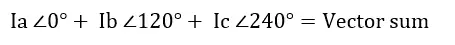# Vector Sum Three Phase Current

### Vector Sum Three Phase Current:

Vector sum is nothing but a calculating total current magnitude as well as phasor. i.e. consider three phase current magnitude and its phasor angle Ia ∠0°, Ib ∠120°, and Ic ∠240°. Here, for calculating vector sum of these current we should effectively consider magnitude as well as phasor quantity of the currents.